What's In a Class? ...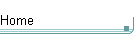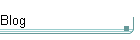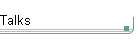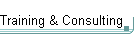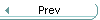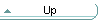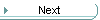On theblogNovember 4: Other Concurrency Sessions at PDC November 3 October 26: Hoare on Testing October 23This is the original article substantially as first published. See the book Exceptional C++ (Addison-Wesley, 2000) for the most current version of this article. The versions in the book have been revised and expanded since their initial appearance in print. The book versions also incorporate corrections, new material, and conformance to the final ANSI/ISO C++ standard.

What's In a Class? - The Interface Principle

I'll start off with a deceptively simple question:

o         What's in a class? That is, what is "part of" a class and its interface?

The deeper questions are:

o         How does this answer fit with C-style object-oriented programming?

o         How does it fit with C++'s Koenig lookup? with the Myers Example? (I'll describe both.)

o         How does it affect the way we analyze class dependencies and design object models?

So, "What's In a Class?"

First, recall a traditional definition of a class:

Class (definition)
A class describes a set of data along with the functions that operate on that data.

Programmers often unconsciously misinterpret this definition, saying instead: "Oh yeah, a class, that's what appears in the class definition -- the member data and the member functions." But that's not the same thing, because it limits the word "functions" to mean just "member functions." Consider:

//*** Example 1 (a)

class X { /*...*/ };

/*...*/

void f( const X& );

The question is: Is f part of X? Some people will automatically say "No" because f is a nonmember function (or "free function"). Others might realize something fundamentally important: If the Example 1 (a) code appears together in one header file, it is not significantly different from:

//*** Example 1 (b)

class X { /*...*/
public:
void f() const;
};

Think about this for a moment. Besides access rights, f is still the same, taking a pointer/reference to X. The this parameter is just implicit in the second version, that's all. So, if Example 1 (a) all appears in the same header, we're already starting to see that even though f is not a member of X, it's nonetheless strongly related to X. I'll show what exactly that relationship is in the next section.

On the other hand, if X and f do not appear together in the same header file, then f is just some old client function, not a part of X (even if f is intended to augment X). We routinely write functions with parameters whose types come from library headers, and clearly our custom functions aren't part of those library classes.

The Interface Principle

With that example in mind, I'll propose the Interface Principle:

The Interface Principle
For a class X, all functions, including free functions, that both
(a) "mention" X, and
(b) are "supplied with" X
are logically part of X, because they form part of the interface of X.

By definition every member function is "part of" X:

(a)  every member function must "mention" X (a nonstatic member function has an implicit this parameter of type X* or const X*; a static member function is in the scope of X); and

(b)  every member function must be "supplied with" X (in X's definition).

Applying the Interface Principle to Example 1 (a) gives the same result as our original analysis: Clearly, f mentions X. If f is also "supplied with" X (for example, if they come in the same header file and/or namespace), then according to the Interface Principle f is logically part of X because it forms part of the interface of X.

So the Interface Principle is a useful touchstone to determine what is really "part of" a class. Do you find it unintuitive that a free function should be considered part of a class? Then let's give real weight to this example by giving a more common name to f:

//*** Example 1 (c)

class X { /*...*/ };

/*...*/

ostream& operator<<( ostream&, const X& );

Here the Interface Principle's rationale is perfectly clear, because we understand how this particular free function works: If operator<< is "supplied with" X (for example, in the same header and/or namespace), then operator<< is logically part of X because it forms part of the interface of X. That makes sense even though the function is a nonmember, because we know that it's common practice for a class' author to provide operator<<. If instead operator<< comes, not from X's author, but from client code, then it's not part of X because it's not "supplied with" X.

Class (definition)
A class describes a set of data along with the functions that operate on that data.

That definition is exactly right, for it doesn't say a thing about whether the "functions" in question are members or not.

Is the IP an OO Principle, or Just a C++-Specific Principle?

I've been using C++ terms like "namespace" to describe what "supplied with" means, so is the IP C++-specific? Or is it a general OO principle that can apply in other languages?

Consider a familiar example from another (in fact, a non-OO) language: C.

/*** Example 2 (a) ***/

struct _iobuf { /*...data goes here...*/ };
typedef struct _iobuf FILE;

FILE* fopen ( const char* filename,
const char* mode );
int   fclose( FILE* stream );
int   fseek ( FILE* stream,
long  offset,
int   origin );
long  ftell ( FILE* stream );
/* etc. */

This is the standard "handle technique" for writing OO code in a language that doesn't have classes: You provide a structure that holds the object's data, and functions -- necessarily nonmembers -- that take or return pointers to that structure. These free functions construct (fopen), destroy (fclose), and manipulate (fseek, ftell, etc.) the data.

This technique has disadvantages (for example, it relies on client programmers to refrain from fiddling with the data directly), but it's still "real" OO code -- after all, a class is "a set of data along with the functions that operate on that data." In this case of necessity the functions are all nonmembers, but they are still part of the interface of FILE.

Now consider an "obvious" way to rewrite Example 2 (a) in a language that does have classes:

//*** Example 2 (b)

class FILE {
public:
FILE( const char* filename,
const char* mode );
~FILE();
int  fseek( long offset, int origin );
long ftell();
/* etc. */
private:
/*...data goes here...*/
};

The FILE* parameters have just become implicit this parameters. Here it's clear that fseek is part of FILE, just as it was in Example 2 (a) even though there it was a nonmember. We can even merrily make some functions members and some not:

//*** Example 2 (c)

class FILE {
public:
FILE( const char* filename,
const char* mode );
~FILE();
long ftell();
/* etc. */
private:
/*...data goes here...*/
};

int fseek( FILE* stream,
long  offset,
int   origin );

It really doesn't matter whether or not the functions are members. As long as they "mention" FILE and are "supplied with" FILE, they really are part of FILE. In Example 2 (a), all of the functions were nonmembers because in C they have to be. Even in C++, some functions in a class' interface have to be (or should be) nonmembers: operator<< can't be a member because it requires a stream as the left-hand argument, and operator+ shouldn't be a member in order to allow conversions on the left-hand argument.

Introducing Koenig Lookup

The Interface Principle makes even more sense when you realize that it does exactly the same thing as Koenig lookup. Here, I'll use two examples to illustrate and define Koenig lookup. In the next section, I'll use the Myers Example to show why this is directly related to the Interface Principle.

Here's why we need Koenig lookup, using an example right out of the standards document:

//*** Example 3 (a)

namespace NS {
class T { };
void f(T);
}

NS::T parm;

int main() {
f(parm);    // OK: calls NS::f
}

Pretty nifty, isn't it? "Obviously" the programmer shouldn't have to explicitly write NS::f(parm), because just f(parm) "obviously" means NS::f(parm), right? But what's obvious to us isn't always obvious to a compiler, especially considering that there's nary a "using" in sight to bring the name f into scope. Koenig lookup lets the compiler do the right thing.

Here's how it works: Recall that "name lookup" just means that, whenever you write a call like "f(parm)", the compiler has to figure out which function named f you want. (With overloading and scoping there could be several functions named f.) Koenig lookup says that, if you supply a function argument of class type (here parm, of type NS::T), then to find the function name the compiler is required to look, not just in the usual places like the local scope, but also in the namespace (here NS) that contains the argument's type. And so Example 3 (a) works: The parameter being passed to f is a T, T is defined in namespace NS, and the compiler can consider the f in namespace NS -- no fuss, no muss.

It's good that we don't have to explicitly qualify f, because sometimes we can't easily qualify a function name:

//*** Example 3 (b)

#include <iostream>
//   declares the free function
//   std::operator<< for strings

int main() {
std::string hello = "Hello, world";
std::cout << hello; // OK: calls
}                     //   std::operator<<

Here the compiler has no way to find operator<< without Koenig lookup, because the operator<< we want is a free function that's made known to us only as part of the string package. It would be disgraceful if the programmer were forced to qualify this function name, because then the last line couldn't use the operator naturally. Instead, we would have to write either "std::operator<<( std::cout, hello );" or "using namespace std;". If those options send shivers down your spine, you understand why we need Koenig lookup.

Summary: If in the same namespace you supply a class and a free function that mentions that class, the compiler will enforce a strong relationship between the two. And that brings us back to the Interface Principle, because of the Myers Example:

More Koenig Lookup: The Myers Example

Consider first a (slightly) simplified example:

//*** Example 4 (a)

namespace NS { // typically from some
class T { }; //   header T.h
}

void f( NS::T );

int main() {
NS::T parm;
f(parm);     // OK: calls global f
}

Namespace NS supplies a type T, and the outside code provides a global function f that happens to take a T. This is fine, the sky is blue, the world is at peace, and everything is wonderful.

Time passes. One fine day, the author of NS helpfully adds a function:

//*** Example 4 (b)

namespace NS { // typically from some
class T { }; //   header T.h
void f( T ); // <-- new function
}

void f( NS::T );

int main() {
NS::T parm;
f(parm);     // ambiguous: NS::f
}              //   or global f?

Adding a function in a namespace scope "broke" code outside the namespace, even though the client code didn't write using to bring NS's names into its scope! But wait, it gets better -- Nathan Myers pointed out the following interesting behaviour with namespaces and Koenig lookup:

//*** The Myers Example: "Before"

namespace A {
class X { };
}

namespace B {
void f( A::X );
void g( A::X parm ) {
f(parm);   // OK: calls B::f
}
}

This is fine, the sky is blue, etc. One fine day, the author of A helpfully adds another function:

//*** The Myers Example: "After"

namespace A {
class X { };
void f( X ); // <-- new function
}

namespace B {
void f( A::X );
void g( A::X parm ) {
f(parm);   // ambiguous: A::f or B::f?
}
}

"Huh?" you might ask. "The whole point of namespaces is to prevent name collisions, isn't it? But adding a function in one namespace actually seems to 'break' code in a completely separate namespace." True, namespace B's code seems to "break" merely because it mentions a type from A. B's code didn't write a using namespace A; anywhere. It didn't even write using A::X;.

This is not a problem, and B is not "broken." This is in fact exactly what should happen. If there's a function f(X) in the same namespace as X, then, according to the Interface Principle, f is part of the interface of X. It doesn't matter a whit that f happens to be a free function; to see clearly that it's nonetheless logically part of X, again just give it another name:

//*** Restating the Myers Example: "After"

namespace A {
class X { };
ostream& operator<<( ostream&, const X& );
}

namespace B {
ostream& operator<<( ostream&, const A::X& );
void g( A::X parm ) {
cout << parm; // ambiguous:
}               //   A::operator<< or
}                 //   B::operator<<?

How Strong Is the "Part Of" Relationship?

While the Interface Principle states that both member and nonmember functions can be logically "part of" a class, it doesn't claim that members and nonmembers are equivalent. For example, member functions automatically have full access to class internals whereas nonmembers only have such access if they're made friends. Likewise for name lookup, including Koenig lookup, the C++ language deliberately says that a member function is to be considered more strongly related to a class than a nonmember:

//*** NOT the Myers Example
namespace A {
class X { };
void f( X );
}

class B {
// class, not namespace
void f( A::X );
void g( A::X parm ) {
f(parm); // OK: B::f,
// not ambiguous
}
};

Now that we're talking about a class B, rather than a namespace B, there's no ambiguity: When the compiler finds a member named f, it won't bother trying to use Koenig lookup to find free functions.

So in two major ways -- access rules and lookup rules -- even when a function is "part of" a class according to the Interface Principle, a member is more strongly related to the class than a nonmember.

If client code supplies a function which mentions X and matches the signature of one provided in the same namespace as X, the call should be ambiguous. B should be forced to say which competing function it means, its own or that supplied with X. This is exactly what we should expect given the IP:

The Interface Principle
For a class X, all functions, including free functions, that both
(a) "mention" X, and
(b) are "supplied with" X
are logically part of X, because they form part of the interface of X.

In short, it's no accident that the Interface Principle works exactly the same way as Koenig lookup. Koenig lookup works the way that it does fundamentally because of the Interface Principle.

(The box "How Strong Is the Relationship?" shows why a member function is still more strongly related to a class than a nonmember.)

What Does a Class Depend On?

"What's in a class?" isn't just a philosophical question. It's a fundamentally practical question, because without the correct answer we can't properly analyze class dependencies.

To demonstrate this, consider a seemingly unrelated problem: Whatoperator<< for a class? There are two main ways, both of which involve tradeoffs. I'll analyze both, and in the end we'll find that we're back to the Interface Principle and that it has given us important guidance to analyze the tradeoffs correctly.

Here's the first way:

//*** Example 5 (a) -- nonvirtual streaming

class X {
/*...ostream is never mentioned here...*/
};

ostream& operator<<( ostream& o, const X& x ) {
/* code to output an X to a stream */
return o;
}

Here's the second:

//*** Example 5 (b) -- virtual streaming

class X { /*...*/
public:
virtual ostream& print( ostream& o ) {
/* code to output an X to a stream */
return o;
}
};

ostream& operator<<( ostream& o, const X& x ) {
return x.print();
}

Assume that in both cases the class and the function appear in the same header and/or namespace. Which one would you choose? What are the tradeoffs? Historically, experienced C++ programmers have analyzed these options this way:

o         Option (a)'s advantage [we is that X has fewer dependencies. Because no member function of X mentions ostream, X does not [appear to] depend on ostream. Option (a) also avoids the overhead of an extra virtual function call.

o         Option (b)'s advantage is that any DerivedX will also print correctly, even when an X& is passed to operator<<.

This analysis is flawed. Armed with the Interface Principle, we can see why -- the first advantage in Option (a) is a phantom, as indicated by the comments in italics:

1.       According to the IP, as long as operator<< both "mentions" X (true in both cases) and is "supplied with" X (true in both cases), it is logically part of X.

2.       In both cases operator<< mentions ostream, so operator<< depends on ostream.

3.       Since in both cases operator<< is logically part of X and operator<< depends on ostream, therefore in both cases X depends on ostream.

So what we've traditionally thought of as Option (a)'s main advantage is not an advantage at all -- in both cases X still in fact depends on ostream anyway! If, as is typical, operator<< and X appear in the same header X.h, then both X's own implementation module and all client modules that use X physically depend on ostream and require at least its forward declaration in order to compile.

With Option (a)'s first advantage exposed as a phantom, the choice really boils down to just the virtual function call overhead. Without applying the Interface Principle, though, we would not have been able to as easily analyze the true dependencies (and therefore the true tradeoffs) in this common real-world example.

Bottom line, it's not always useful to distinguish between members and nonmembers, especially when it comes to analyzing dependencies, and that's exactly what the Interface Principle implies.

Some Interesting (and Even Surprising) Results

In general, if A and B are classes and f(A,B) is a free function:

o         If A and f are supplied together, then f is part of A and so A depends on B.

o         If B and f are supplied together, then f is part of B and so B depends on A.

o         If A, B, and f are supplied together, then f is part of both A and B, and so A and B are interdependent. This has long made sense on an instinctive level... if the library author supplies two classes and an operation that uses both, the three are probably intended to be used together. Now, however, the Interface Principle has given us a way to rigorously prove this interdependency.

Finally, we get to the really interesting case. In general, if A and B are classes and A::g(B) is a member function of a:

o         Because A::g(B) exists, clearly A always depends on B. No surprises so far.

o         If A and B are supplied together, then of course A::g(B) and B are supplied together. Therefore, because A::g(B) both "mentions" B and is "supplied with" B, then according to the Interface Principle it follows (perhaps surprisingly, at first!) that A::g(B) is part of B and, because A::g(B) uses an (implicit) A* parameter, B depends on A. Because A also depends on B, this means that A and B are interdependent.

At first, it might seem like a stretch to consider a member function of one class as also part of another class, but this is only true if A and B are also supplied together. Consider: If A and B are supplied together (say, in the same header file) and A mentions B in a member function like this, "gut feel" already usually tells us A and B are probably interdependent. They are certainly strongly coupled and cohesive, and the fact that they are supplied together and interact means that: (a) they are intended to be used together, and (b) changes to one affect the other.

The problem is that, until now, it's been hard to prove A and B's interdependence with anything more substantial than "gut feel." Now their interdependence can be demonstrated as a direct consequence of the Interface Principle.

Note that, unlike classes, namespaces don't need to be declared all at once, and what's "supplied together" depends on what parts of the namespace are visible:

//*** Example 6 (a)

//---file a.h---
namespace N { class B; } // forward decl
namespace N { class A; } // forward decl
class N::A { public: void g(B); };

//---file b.h---
namespace N { class B { /*...*/ }; }

Clients of A include a.h, so for them A and B are supplied together and are interdependent. Clients of B include b.h, so for them A and B are not supplied together.

Summary

I'd like you to take away three thoughts:

o         The Interface Principle: For a class X, all functions, including free functions, that both (a) "mention" X, and (b) are "supplied with" X are logically part of X, because they form part of the interface of X.

o         Therefore both member and nonmember functions can be logically "part of" a  class. A member function is still more strongly related to a class than is a nonmember, however.

o         In the Interface Principle, a useful way to interpret "supplied with" is "appears in the same header and/or namespace." If the function appears in the same header as the class, it is "part of" the class in terms of dependencies. If the function appears in the same namespace as the class, it is "part of" the class in terms of object use and name lookup.

Notes

1. Even those may be unchanged if the original f was a friend.

2. We'll examine the relationship with namespaces in detail later in the article, because it turns out that this Interface Principle acts exactly the same way as Koenig lookup.

3. The similarity between member and nonmember functions is even stronger for certain other overloadable operators. For example, when you write "a+b" you might be asking for a.operator+(b) or operator+(a,b), depending on the types of a and b.

4. Named after Andrew Koenig, who nailed down its definition and is a longtime member of both AT&T's C++ team and the C++ standards committee. See also A. Koenig and B. Moo, Ruminations on C++ (Addison-Wesley, 1997).

5. There's a little more to the mechanics, but that's essentially it.

6. By value, reference, pointer, or whatever.

7. Granted, that relationship is still less strong than the relationship between a class and one of its member functions. See the box "How Strong Is the Relationship?" later in this article.

8. Nathan is another longtime member of the C++ standards committee, and the primary author of the standard's locale facility.

9. This specific example arose at the Morristown meeting in November 1997, and it's was what got me thinking about this issue of membership and dependencies. What the Myers Example means is simply that namespaces aren't quite as independent as people originally thought, but they are still pretty independent and they fit their intended uses.